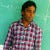# Split an array into two equal Sum subarrays

This question asked in Facebook and Google and many more good tech company's….

Given an array of integers greater than zero, find if it is possible to split it in two subarrays (without reordering the elements)

Examples :

`Input : Arr[] = { 1 , 2 , 3 , 4 , 5 , 5  }Output :  { 1 2 3 4 }           { 5 , 5 }Input : Arr[] = { 4, 1, 2, 3 }Output : {4 1}         {2 3}Input : Arr[] = { 4, 3, 2, 1}Output : Not Possible...`

Approch-1 :- Brute-Force…

# Two Sum

Given an array of integers `nums` and an integer `target`, return indices of the two numbers such that they add up to `target`.

You may assume that each input would have exactly one solution, and you may not use the same element twice.

Example 1:

`Input: nums = [2,7,11,15], target = 9Output: [0,1]Output: Because nums + nums == 9, we return [0, 1].`

Example 2:

`Input: nums = [3,2,4], target = 6Output: [1,2]`

Example 3:

`Input: nums = [3,3], target = 6Output: [0,1]`

Approach-1 :- Brute Force__ Time: O(n²) & Space: O(1)

`public int[] twoSum(int[] nums…`

# GOLang Slices Guide

Slices are one of the powerful and flexible concept than arrays in go-language.

It is a light weight data structure.

Slice is an abstraction over an array and overcomes limitations of arrays. Like getting a size dynamically or creating a sub-array of its own and hence much more convenient to use than traditional arrays

Slices are allow to store same type of data, you are not allowed to store different types of data elements in same slice.

The main difference between slice and array in go lang is arrays are fixed in size slice are re-sizable.

Internally, slice and an…

# Find maximum sum path by involving elements from two arrays

The idea is to do something similar to merge process of merge sort. This involves calculating the sum of elements between all common points of both arrays. Whenever there is a common point, compare the two sums and add the maximum of two to the result.

Input: X = { 3, 6, 7, 8, 10, 12, 15, 18, 100 } Y = { 1, 2, 3, 5, 7, 9, 10, 11, 15, 16, 18, 25, 50 } The maximum sum path is: 1 → 2 → 3 → 6 → 7 → 9 → 10 → 12 → 15 →…

# Balanced Parentheses

This the frequently asked question in FANG & Top Rated Leet Code Problem.. solution in Java.

Approach-1 : Time Complexity : O(n) & Space: O(n).

This problem is to check how much good you are at data structures. Best Data Structure to solve this problem is Stack or ArrayDeque(In Java) faster than Stack. To validate current char I used switch-case instead of if-else

`import java.util.Stack;/** * * @author SivaReddy Uppathi */public class BalancedParathesis {// Approch-1 private static boolean isBalanced(String expression) { // ArrayDeque is faster than Stack class // Deque<Character> stack = new ArrayDeque<>(); Stack<Character> paranthasis =…`

# Check whether two strings are anagram of each other or not

This the frequently asked question in FANG & All Top campiness.. solution in Java.

Approach-1: (By Using Sorting Method) : Time Complexity : O(nlog(n))

`package chalanges.compitative;import java.util.Arrays;/** * * @author SivaReddy Uppathi */public class Anagram {public static void main(String[] args) {        char str1[] = {'s', 'i', 'v', 'a'};        char str2[] = {'s', 'v', 'a', 'i'};        boolean isAnagram = checkAnagramUsingSorting(str1, str2);        System.out.println(isAnagram);}private static boolean checkAnagramUsingSorting(char[] str1, char[] str2) {        if (str1.length != str2.length) {            return false;        }        Arrays.sort(str1);        Arrays.sort(str2);        for (int i = 0; i < str1.length; i++) {            if (str1[i] != …`## Sivareddy Uppathi

Full Stack Software Developer| Programming enthusiast | Loves DS-Algo and System Design